# Inverse Functions (3 Key Things To Remember)

When you reach a certain point in your math career, perhaps around 8th or 9th grade, you likely learned about the Vertical Line Test, which is a test that determines if a relation is a function. Further down the road, you’ve likely learned about inverse functions. This is a pretty big topic in math, and it’s the focus of this article. Let’s get started!

Reminder:

A relation is a function if it passes the Vertical Line Test. To test if a relation is a function, imagine dropping a vertical line through any x value.

If any such vertical line crosses the graph at more than one point, the relation fails the Vertical Line Test. It is NOT a function. If every vertical line that is drawn through the graph hits no more than one point, the relation passes the Vertical Line Test. It is a function.

## What is an inverse function?

An easy way to think about inverse functions is to go back to elementary school math. Yes, that’s right, let’s think about when we learned how addition and subtraction are inverse operations.

That is, adding 5 to a number is the opposite, or inverse, of subtracting 5 from a number. You may even remember that 5 and -5 are called additive inverses. Adding them together gives us the identity for addition: 0. The two operations undo each other.

In a similar way, multiplication and division are inverse operations. Multiplying a number by 6 is the inverse operation of dividing by 6 (or multiplying by the reciprocal ⅙). If we multiply 6 by ⅙, we obtain 1, the identity for multiplication.

The idea of inverses carries over to functions as well. An inverse function will undo the result of a function.

Inverse functions relate to the composition of functions. A simple way to think of it – if a function takes each input and adds 10 to it, the inverse function subtracts 10.

More generally, if the inputs x of a function f produce outputs y, then putting the y values as inputs into the inverse function will produce the outputs x. Did you get that? The diagram above may help.

The inputs of the original function become the outputs of the inverse function and the outputs of the original function become the inputs of the inverse function. The domain and the range switch! If a point (x, y) is on the original function, then the point (y, x) is on the inverse function.

## How can we tell if the inverse of a function will also be a function?

There is a convenient test that helps us determine if the inverse of a function is also a function. Just as the Vertical Line Test tells us if the original relation is a function, the Horizontal Line Test helps us determine if the inverse of a function will also be a function.

Let’s think about this a bit. The original function passes the Vertical Line Test. As we mentioned, this means that for each input, there is exactly one output. Since an inverse relation switches the domain and the range, for the inverse to be a function it would have to also pass the vertical line test.

Thinking about the original function though, we can use the Horizontal Line Test to determine if the inverse will be a function.

## Examples of graphs that pass/fail the Horizontal Line Test (HLT):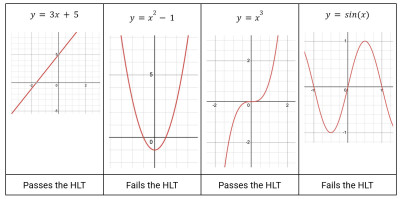Here are some functions that either pass or fail the horizontal line test (the ones that fail are not one to one functions).

## How do we find the inverse of a function from a graph?

To find the inverse of a function from a graph we reflect the function f(x) over the identity function y=x to obtain the graph of the inverse f-1(x).

### Example

Consider the function f(x) = x3 – 2.  A quick look at the graph on Desmos shows us a few key points on this function :(-1, -3) (0, -2), and (1, -1), and . Also, notice that the function passes the Horizontal Line Test, which guarantees the inverse will also be a function.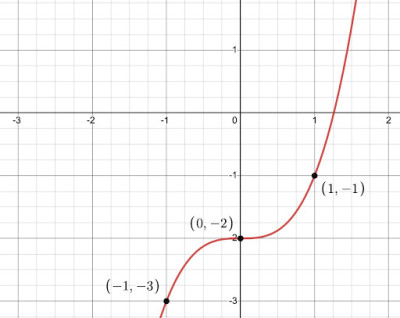The graph of the function f(x) = x3 – 2 with a few key points marked.

To find the inverse of the function, we reflect our function over the identity function y=x. This has the effect of switching the x and y coordinates of the ordered pairs.

Notice when we switch up the ordered pairs, the new ordered pairs are on the inverse function!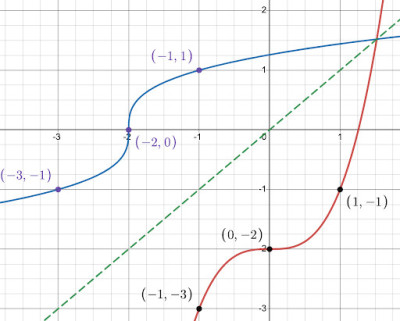To find the inverse (blue curve) of the function f(x) = x3 – 2 (red curve), we reflect its graph across the line y = x.

Note: The red graph is the original function f(x) = x3 – 2, the green dashed line is the identity function y=x, and the blue graph is the inverse function f-1(x) = 3√(x+2). The inverse function is the reflection of the original function over the identity function.

## How do we find the formula for an inverse function?

There is a relatively simple process for finding the equation of an inverse function. Since the inputs of the original function become the outputs of the inverse function, we can switch our domain and range to find the inverse function.

In practice, this means we can switch the x and y coordinates, then solve for the “new” y

### Example

If f(x) = 2x-5 write the equation of the inverse function f-1(x).

Solution

Step 1: Replace f(x) with the variable y, which will make the equations easier to work with.

f(x) = 2x-5

y = 2x-5

Step 2: Switch the variables x and y. Think about this: if the point (x, y) is on the original function, then the point (y, x) is on the inverse function.

x = 2y-5

Step 3: Solve for y. The resulting function will be the inverse function!

x = 2y-5

x+5 = 2y

(x+5)/2 = y

y= (x+5)/2

Step 4: Replace y with f-1(x).

f-1(x)= (x+5)/2

And there we have it!

## How can we check to see if our formula for an inverse function is correct?

We can easily check if our formula for the inverse is correct by testing the function compositions. Since the inverse functions undo each other, when we compose f-1(x) with f(x) then the result should be x, which is the identity function. Because function composition is not commutative (that is, changing the order of the function composition can change the result) we need to check both function compositions listed below.

### Example

If f(x)=2x-1, find f-1(x). Verify that f and f-1 are inverses.

Solution

To find the inverse function for f(x)=2x-1, or y=2x-1, we follow the steps above. Start by switching the domain x and the range y. Then solve for y; this will be the inverse function.

y=2x-1

x=2y – 1

x+1=2y

(x+1)/2=y

f-1(x)=(x+1)/2

Check:

f-1(f(x)) =f-1(2x-1)

Now, plug 2x-1 into the inputs x of the inverse function:

=((2x-1)+1)/2

=(2x-1+1)/2

=2x/2

=x

Great! So, it looks as though we have the correct inverse function. We still need to show that f(f-1(x))=x.

f(f-1(x)) = f((x+1)/2)

Now, we plug x+12 into the input for the f function:

=2((x+1)/2) -1

Cancel the 2s:

=x+1-1

=x

Terrific! We have now shown that our inverse function is correct!

## What about functions that don’t pass the Horizontal Line Test? Is there anything we can do to fix this?

There are many situations in which we’d like to talk about the inverse of a function, even if the original function doesn’t pass the Horizontal Line Test. For example, the parabola y = x2 fails the Horizontal Line Test, so the inverse isn’t a function.

A quick glance at the graph shows us that the parabola, when reflected over the identity function y=x gives us a parabola on its side, which fails the Vertical Line Test!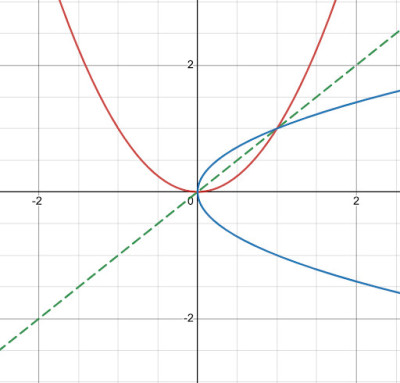A parabola (red) is not a one-to-one function, so its domain must be restricted for it to have a well-defined inverse.

Mathematicians deal with this problem by restricting the domain of the original function to a portion of the graph that is one to one, that is, that will pass the HLT.

An obvious choice for restricting our domain of the original parabola is to define the function y = x2 on the interval  (0, ∞). Now our graph will pass the HLT: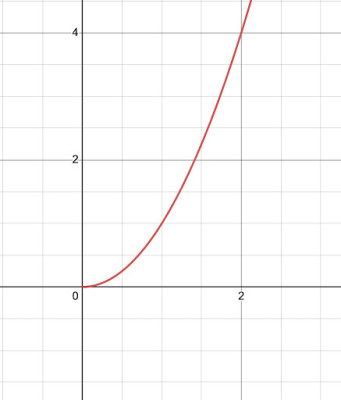This is the graph of y = x2 with a domain restriction of x >= 0. This gives us a one-to-one function.

This means, when we sketch the inverse, it too will be a function, as shown below.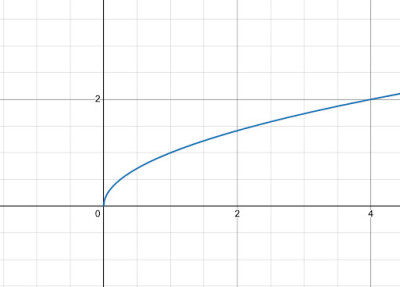This is the graph of y = √x, which is the inverse of y = x2 on the domain x >= 0.

Inverse functions are a big topic! Hopefully you gained a solid foundation on how they work from this article. For further exploration, consider how you would restrict the domain of the y=sin x and y=cos x in order to guarantee that the inverses will also be functions.